# Ignition vs Gazebo

Since Gazebo 11 will be the last major version I thought I’d test the replacement Ignition. There’s a handy comparison chart with feature comparisons between the two programs.

But how does it actually perform?

The following tests compare the output of Gazebo 11 and Ignition Citadel.

The salient differences between the two simulators are as follows:

Gazebo 11:

• Physics: ODE
• Visualization: OGRE

• Physics: DART
• Visualization: OGRE*

*By default, Ignition supports OGRE2, this is incompatible with my system, so I’ve opted for OGRE visualizations

Let’s take a look!

## Inertia

Inertia is an important element in simulation, as it lets the physics simulator calculate the correct dynamics of an object in simulation.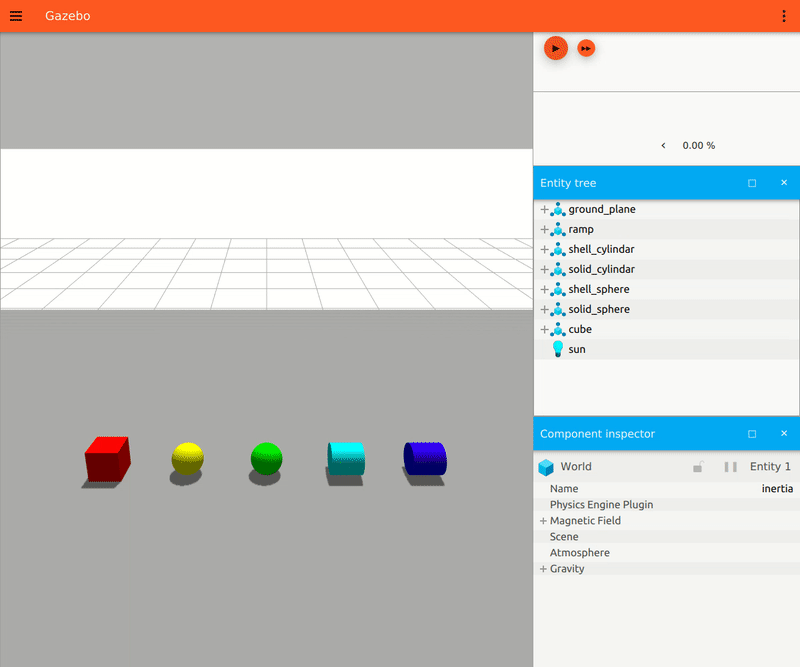From left to right: cube, solid sphere, hollow sphere, solid cylinder, hollow cylinder. All shapes have the same mass and are modeled with high friction. The high friction causes the cube to stay in place, while the other shapes are able to roll down the ramp as expected.

Nothing surprising here! Both Ignition and Gazebo calculate the dynamics of inertia correctly.The only noticeable difference is the rendering. The Gazebo version looks much darker even though all lighting properties are the same, and the shapes aren’t showing any shadowing on the ground plane like they are in Ignition.

Remarkably, removing shadowing from Gazebo renders more similarly to the Ignition version.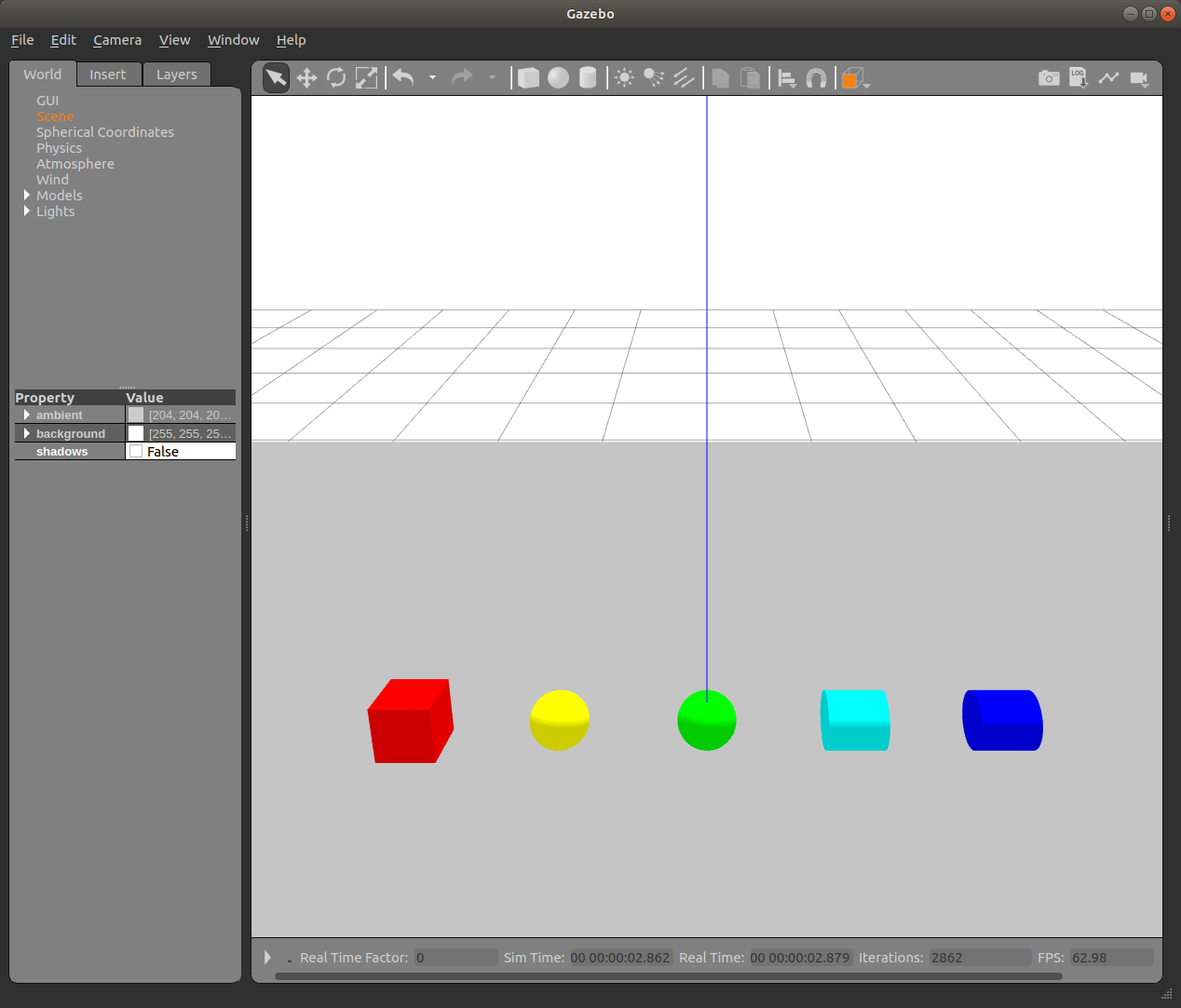## Friction

Friction is the first place that shows the most significant difference between Gazebo and Ignition’s default physics engines.

There are several different types of friction that you can model. In the sdformat specification, the most common is the coefficient of friction. The coefficient of friction in version 1.7 of the sdformat is modeled as an ODE parameter with `mu` as the coefficient for the “first friction direction” and `mu2` as the coefficient for the “second friction direction”. There is an additional parameter, `fdir1` that can specify a specific primary friction direction relative to the link, otherwise it is modeled relative to the world.

Most notably, neither simulation performed exactly as I thought it would. In the following tests, boxes I expected to move down the ramp are colored green, while boxes that I expect to remain stationary are colored red.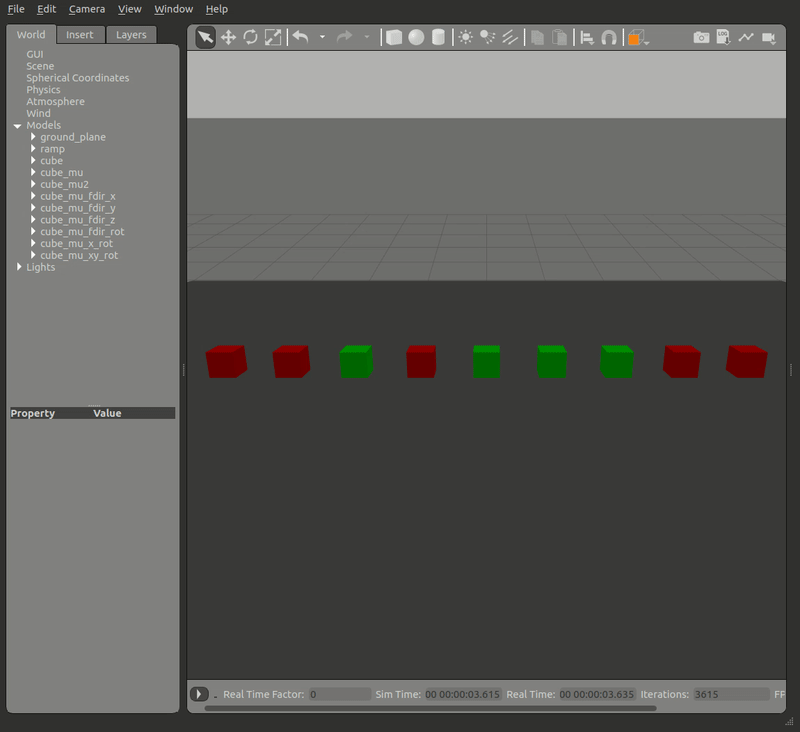From left to right:

Cube1: Default friction. sdformat states that if no friction is set, mu1 and mu2 are set to 1 (high friction). Therefore, this cube shouldn’t move.

Cube2: `mu=1`, `mu2=0`. The ramp is pointed down the `X` axis. Therefore, I expect that with a high mu along that axis, the cube will not move

Cube3: `mu=0`, `mu2=1`. The ramp is pointed down the `X` axis. Therefore, I expect that with no friction along this direction, the cube will move down the ramp.

Cube4: `mu=1`, `mu2=0`, `fdir1=1 0 0` (pointed down the ramp). Since the mu value is aligned with the ramp, I expect the block to stay still.

Cube5: `mu=1`, `mu2=0`, `fdir1=0 1 0` (pointed toward the sides of the ramp). Since the mu value is perpendicular to the ramp, I expect the block to move.

Cube6: `mu=1`, `mu2=0`, `fdir1=0 0 1` (pointed up). Since the mu value is perpendicular to the ramp, I expect the block to move.

Cube7: Cube4, rotated along the Y axis Since the mu value is rotated to be perpendicular to the ramp, I expect the block to move.

Cube8: `mu=1` rotated along the X axis. Since only setting mu should set both values, rotation shouldn’t matter and the block shouldn’t move.

Cube9: `mu=1` rotated along the X and Y axis. Since only setting mu should set both values, rotation shouldn’t matter and the block shouldn’t move.

What’s neat is that the cubes 6 and 7 tumble on the transition from the ramp to the ground as the high friction face comes in contact with the edge.

In Gazebo, the `mu` and `mu2` arguments acted on the opposite axis as I expected, with the world `y` frame corresponding to `mu` and the world `x` frame corresponding to `mu2`.In Ignition, the `mu` and `mu2` arguments act on the axis that I expected, with `x` corresponding to `mu` and `y` corresponding to `mu2`. However, setting the `fdir1` direction to orientations perpendicular to the ramp direction incorrectly makes the box stay on the ramp.

Delving a little bit deeper into the implementation of the friction pyramid, we see that the x and y axis are indeed flipped.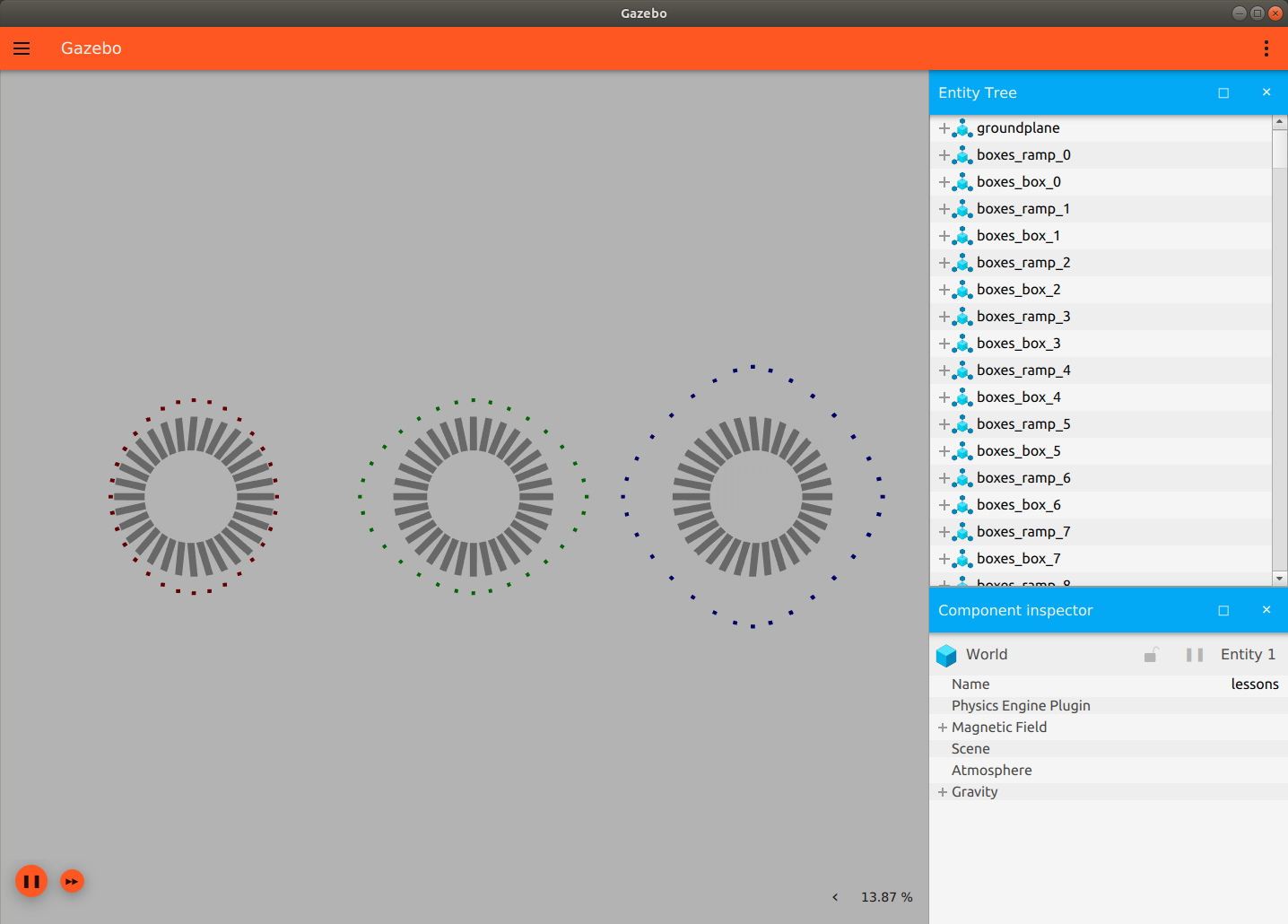You can also clearly see better performance in the Ignition simulation of friction, with Ignition producing much smoother results.

This does, however come at a computational expense. The realtime factor of Gazebo on my computer ranged between [0.3, 0.99], whereas the realtime factor of Ignition hovered at around 0.2.

## Bounce

Bounce is another area with a large difference between the two simulators. It is not currently modeled in Ignition, so if you require bounce Ignition is currently a no-go for you.To model bounce in Gazebo you need to set 3 parameters in the SDF.

1. `restitution_coefficient` [0,1] Where 0 is no bounce, and 1 is pure bounce
2. `threshold` The penetration threshold needed to apply restitution force
3. `max_vel` The maximum velocity that the restitution force can cause.

True infinite bounce is only achieved if the ground also has the `restitution_coefficient` set to 1. Note you can set the `max_vel` of the ground to 0 so it doesn’t accrue any velocity.

## Joint Dynamics

Both joint damping and joint friction behave similarly in both versions.

Joint damping is modeled as viscous damping in units of N*m*s/rad, or the amount of opposing force to any joint velocity (in this case torque per angular velocity) that is used to “slow” a moving joint towards rest.

Damping in Gazebo:Damping in Ignition: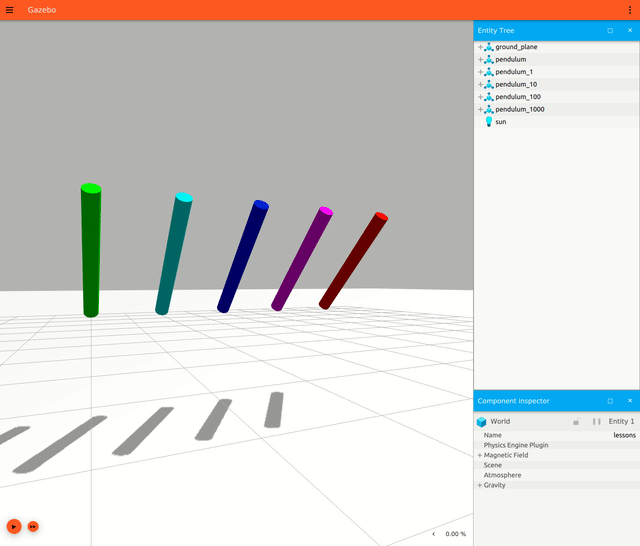From left to right: Green: 0, Turquoise: 1, Blue: 10, Pink: 100, Red: 1000

Joint friction is modeled as kinetic friction with the coefficient of friction that is used to oppose the velocity of a joint to “slow” a moving joint to rest.

Friction in Gazebo:Friction in Ignition:From left to right: Green: 0, Turquoise: 1, Blue: 10, Pink: 100, Red: 1000

I thought it would be good to also model these properties on a wheel, since that is a common use case.

Wheel motion

Gazebo:Ignition:From front to back: Yellow: none, Turquoise: damping, Pink: friction, Green: spring reference/stiffness.

## Conclusions

Your use case will likely determine which version you end up going for. Overall Ignition has some nicer friction properties, but is lacking in some (perhaps less often used) areas such as bounce.

If you have simple simulation needs and want to stay on top of the latest versions, Ignition is probably the right call. Otherwise, you’re probably better off staying with Gazebo, at least for now.

### Summary

attribute Ignition Gazebo
Inertia x x
Friction
Bounce   x
Dynamics x x